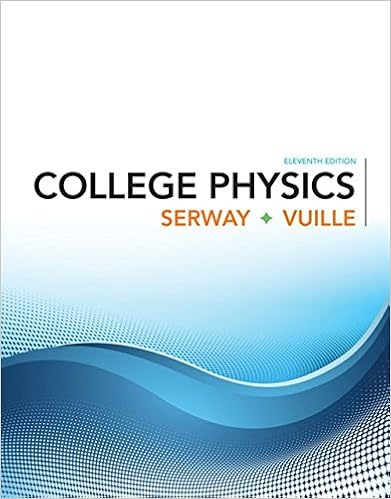# Which of the following lists the substances in order

• Notes
• 5
• 100% (1) 1 out of 1 people found this document helpful

This preview shows page 2 - 4 out of 5 pages.

##### We have textbook solutions for you!
The document you are viewing contains questions related to this textbook.The document you are viewing contains questions related to this textbook.
Chapter 15 / Exercise 15.4
College Physics
Serway/VuilleExpert Verified
6.Which of the following lists the substances in order of increasing strength of intermolecular forces?A.Ne > RbF > PF3> H2OB. PF3< H2O < RbF < NeC.Ne < PF3< H2O < RbFD.RbF < H2O < PF3< NeE. H2O > Ne > PF3> RbF
7.A sample of ammonia gas at 65.5°C and 524 mmHg has a volume of 15.31 L. What is its pressure when the temperature is –15.8°C and its volume is 1.5 L?
8.Assuming ideal behavior, what is the density of argon gas at STP (1 atm, 0°C), in g/L?
9.What is the strongest intermolecular force in trimethylamine?
##### We have textbook solutions for you!
The document you are viewing contains questions related to this textbook.The document you are viewing contains questions related to this textbook.
Chapter 15 / Exercise 15.4
College Physics
Serway/VuilleExpert Verified
10. An unknown liquid is vaporized in a 273 mL flask by immersion in a water bath at 99°C. The barometric pressure is 753 mmHg. If the mass of the vapor retained in the flask is 1.362 g, what is its molar mass?A.154 g/molB. 40.9 g/mol C. 112 g/mol D. 20.4 g/molE. 184 g/mol
11. Linolenic acid (C18H30O2) can be hydrogenated to stearic acid by reacting it with hydrogen gas according to the equation:C18H30O2+ 3H2C18H36OWhat volume of hydrogen gas, measured at STP (1 atm, 0°C), is required to react with 10.5 g of linolenic acid in this reaction?2
422 mL
•••